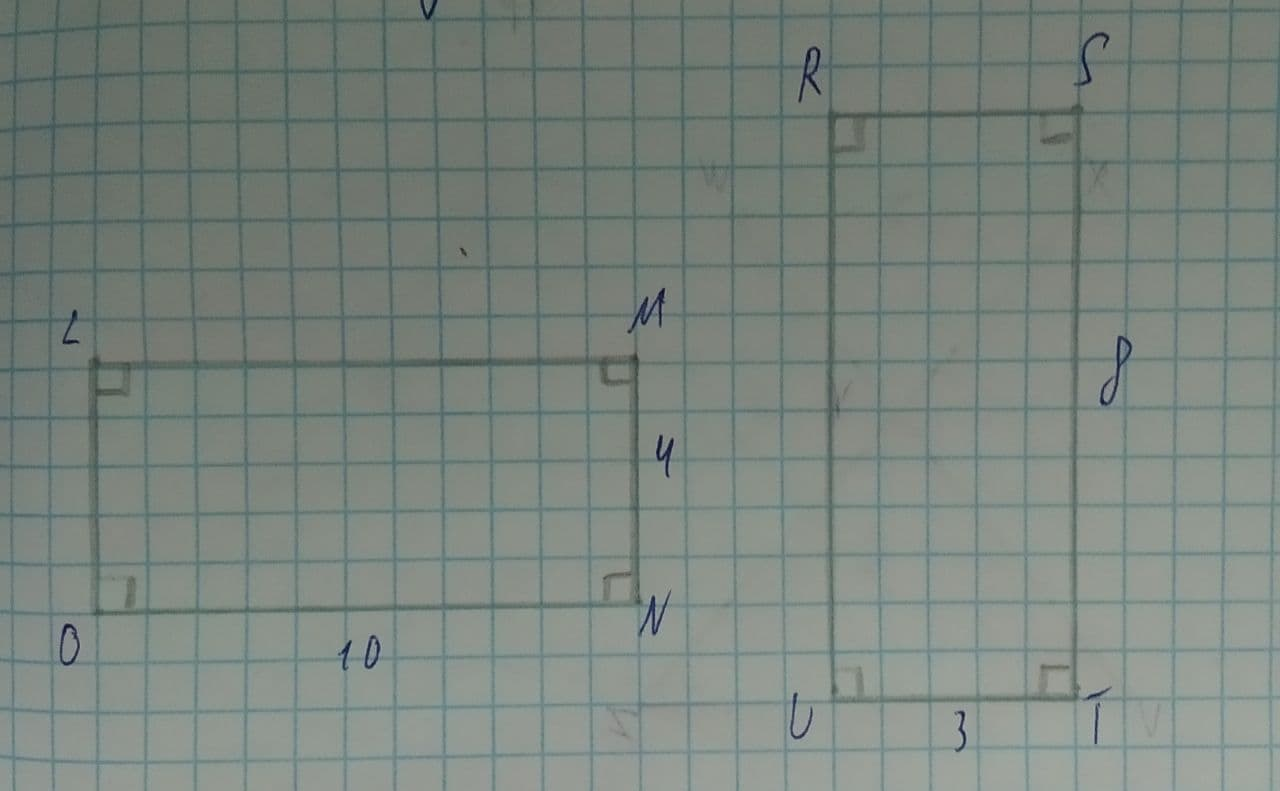# Determine whether the polygons are similar. If so, write a similarity statement and give the scale factor. If not, explain.Joni Kenny 2021-08-11 Answered
Determine whether the polygons are similar. If so, write a similarity statement and give the scale factor. If not, explain.You can still ask an expert for help

• Questions are typically answered in as fast as 30 minutes

Solve your problem for the price of one coffee

• Math expert for every subject
• Pay only if we can solve itstuth1

In the given rectangle LMNO, it is given that MN=4 and ON=10.
In the rectangle RSTU, UT=3 and ST=8.
Rectangles LMNO and RSTU are similar if $\frac{ON}{ST}=\frac{MN}{UT}$
Now,
$\frac{ON}{ST}=\frac{10}{8}$
$=\frac{5}{4}$
$\frac{MN}{UT}=\frac{4}{3}$
$\frac{ON}{ST}\ne \frac{MN}{UT}$
So, rectangles LMNO and RSTU are not similar